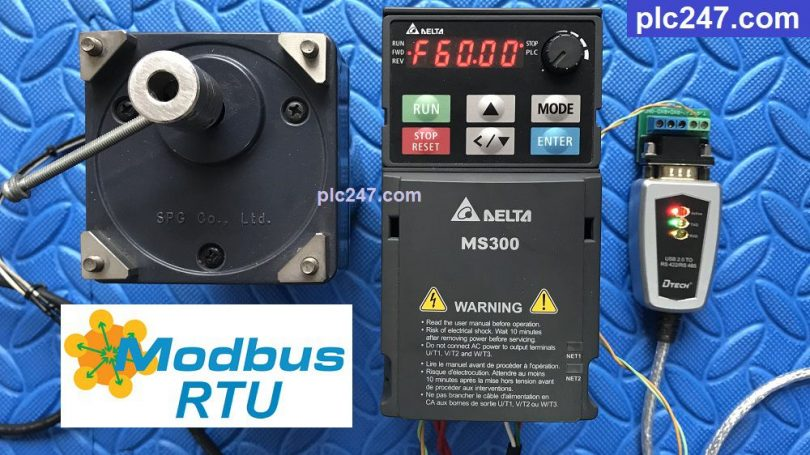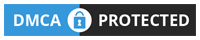# DELTA MS300 “Modbus RTU” via Modbus PollWritten by

Hello everyone! In previous articles, we have used many different types of PLCs to control Delta MS300 inverters via Modbus-RTU RS485 communication standard.

Today plc247.com will guide you to use PC (using USB to RS485 port) to control DELTA MS300 inverter. This is a very fast way, we only need a few minutes to control the inverter as well as store data.

### PC & DELTA MS300 “Wiring Diagram”

You can use any USB to RS485 port

#### DELTA MS300 VFD Communication Setting

In addition to the parameters of the inverter installed according to the motor (frequency, voltage, current), we need to set the communication parameters as follows:

+ P00.20 = 1 (Modbus-RTU Control Frequency)

+ P00.21 = 2 (Modbus-RTU Control Motor)

+ P09.00 = 1 (Address Slave = 1)

+ P09.01 = 9.6 (9600bps)

+ P09.02 = 0

+ P09.03 = 1.0 (Time Detect Err = 1.0s)

+ P09.04 = 14 (Modbus-RTU Slection 8bit / stop bit = 1/ Even)

>>> Motor Control Address = 2000 (hex)

+ Set Word 2000 = 18 (dec): Forward Run
= 34 (dec): Reverse Run
= 1 (dec): Stop Run

>>> Frequency Address = 2001 (hex)

• Output Frequency = 2103 (hex)
• Output Current = 2104 (hex)
• Output Voltage = 2106 (hex)

### “Modbus Poll” Programming

+ Motor Control Command

+ Setting Frequency Command

+ Data Monitoring Command

### Project Tutorial Video

======

Recommended software and documentation